## nJFET Characteristic Curves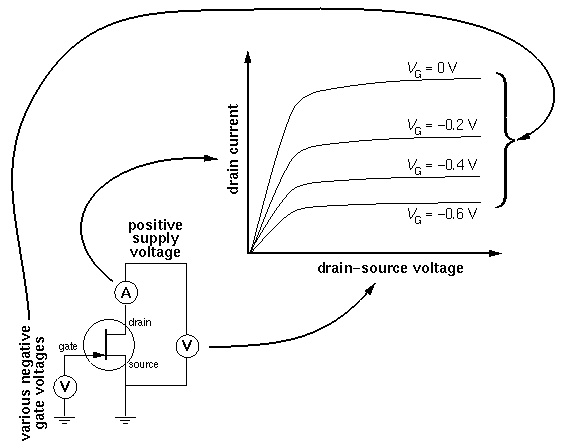#### Measured Characteristic Curves for 2N5457for VG = 0 V, -.2 V, -.4 V, ...., -1.4 V

big data file, smaller data file, postscript plot, pdf plot

The behavior of an n-channel junction field-effect transistor (nJFET) is largely controlled by the voltage at the gate (usually a negative voltage). For the usual drain-source voltage drops (i.e., the saturation region: positive voltages from a few volts up to some breakdown voltage) the drain current (ID) is nearly independent of the drain-source voltage (VDS), and instead depends on the gate voltage (VG). (This is unusual behavior: usually more voltage produces to more current, but here the current only increases slightly with increasing VDS.) The transconductance, i.e., the ratio of the change in drain current to change in gate voltage, often denoted by g or yfs, is

g = yfs =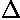ID/VG

In the simplest approximation the characteristic curves of a nJFET are a set of flat lines:Each (flat) curve shows that ID doesn't change with changing VDS. The different levels show that ID does depend on VG. The spacing of the constant-ID curves is usually not constant, instead ID depends quadratically on VG:

ID=K(VG-VTO)2

where the threshold (or pinch-off) voltage VTO and K are constants. The maximum drain current (which occurs at zero-gate voltage, i.e., VG=0) is denoted IDSS. The value of K can be determined from IDSS and VTO:

IDSS = K(0-VTO)2

A slightly more complicated approximation takes into account the sloping of the characteristic curves. In bipolar transistors this is due to the Early Voltage, here a very similar equation results from quite different physics: channel length modulation. The equation looks like:

1/VA =So in this model all the characteristic curves all have a common x-axis intercept at the large negative voltage -1/. (The dashed curves are far from the active region and in no way represent the actual behavior of the transistor for negative VDS. In fact, the transistor is not designed to be operated with negative VDS.)(For the above measured 2N5457, 1/ranges from 250 to 750 V, so the characteristic curves have small but variable slopes.)

The actual relationship between the drain current (ID) and the controlling gate voltage (VG) and drain-source voltage drop (VDS) is some complicated function which we can denote:

ID(VG,VDS)

Like any function we can approximate it near a particular point using just the first terms of a Taylors expansion:Clearly these admittance (y) parameters are not constants. For example yos is the slope of a characteristic curve, which is small for voltages near threshold and increases for larger VG. (Note that slope on an I-V is basically the inverse of the resistance. Thus 1/yos can be described as the output impedance. A typical value for 1/yos would be 100,000.)

The defining parameter -- the transconductance g or yfs -- is not at all constant. To the extent that the drain current depends quadratically on the gate voltage, the transconductance -- which is the derivative: dID/dVG -- depends linearly on VG above threshold. Below is the measured relationship: ID vs VG for the above 2N5457. Notice that for VG>VTO, the drain current is well approximated by the quadratic, whereas for VG<VTO (i.e., sub-threshold) the drain current depends approximately exponentially on VG. Thus the drain current is not strictly zero below threshold, merely exponentially small.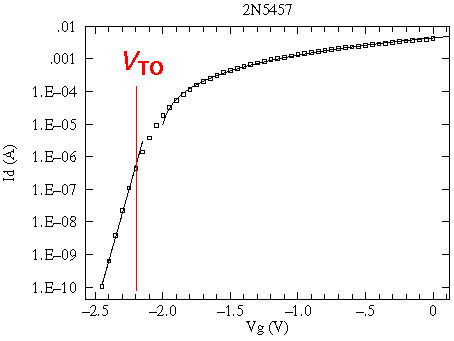The characteristic curves focus on the output of the transistor, but we can also consider the behavior of the input. In normal operation the gate is a reversed biased diode, and so IG is essentially zero (which should sound like a huge input resistance). As a result the outputs have little effect on the inputs, but if we follow the traditional analysis the actual functional relationship giving the gate current (IG) from the gate voltage (VG) and drain-source voltage drop (VDS) is some complicated function which we can denote:

IG(VG,VDS)

Like any function we can again approximate it near a particular point using just the first terms of Taylors expansion: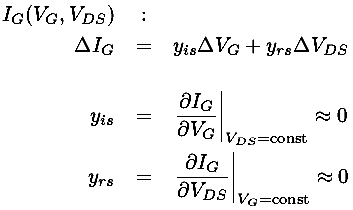The small values of yrs and yis shows that the input is largely unaffected by the output and that the input resistance is huge. In fact this input resistance is so large that the capacitance reactance is almost always of greater significance.

Finally it should be noted that in the small VDS region -- before the saturation region -- the FET characteristic curves look like nearly straight lines through the origin. VG controls the slope of these lines, so the FET acts like a variable resistor with a voltage (VG) control. Here is a plot of this region for the above 2N5457: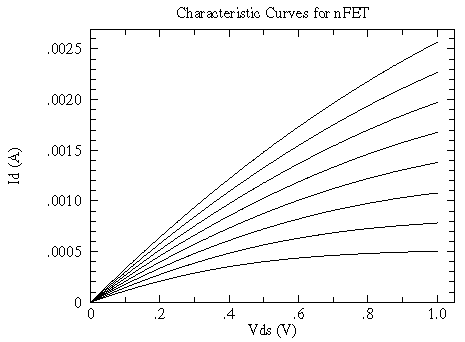The spec sheet reports the following values for the 2N5457:

Characteristics Symbol Min Max Unit
Forward Transfer Admittance yfs 1 5 m mhos
Output Admittance yos - 50 µ mhos
Zero-Gate Voltage Drain Current IDSS 1 5 mA
Gate Source Cutoff Voltage VTO -0.5 -6.0 V
Input Capacitance CiSS - 7.0 pF